# Hydrogenaudio Knowledgebase:Sandbox

Article texta more textb more text c.

• Bulleted textb
• Bulleted textc.
• intervening text
• ^a Text for note a.
• ^b Text for note b.
• ^c Text for note c (with extended highlighting).

lkhjijhglkjh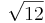$\sqrt{12}$

diff = 10 × log10(P2 / P1)

diff = 10 × log10(p22 / p12)

diff = 20 × log10(p2 / p1)  because log10(a2) = 2 × log10(a)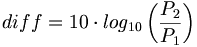${diff} = 10 \cdot log_{10}\left(\frac{P_2}{P_1}\right )$${diff} = 10 \cdot log_{10}\left(\frac{p_2^2}{p_1^2}\right )$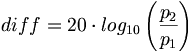${diff} = 20 \cdot log_{10}\left(\frac{p_2}{p_1}\right )$ because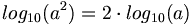$log_{10}(a^2) = 2 \cdot log_{10}(a)$


some text that is not formated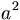$a^2$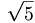$\sqrt{5}$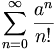$\sum_{n=0}^\infty \frac{a^n}{n!}$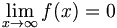$\lim_{x\to\infty}f(x)=0$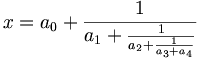$x = a_0 + \frac{1}{a_1 + \frac{1}{a_2 + \frac{1}{a_3 + a_4}}}$

Does things work after update someone wonders... Hope the upgrade works!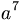$a^7$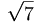$\sqrt{7}$ test testing plugin

Multiplication table
× 1 2 3
1 1 2 3
2 2 4 6
3 3 6 9
4 4 8 12
5 5 10 15

foobar2000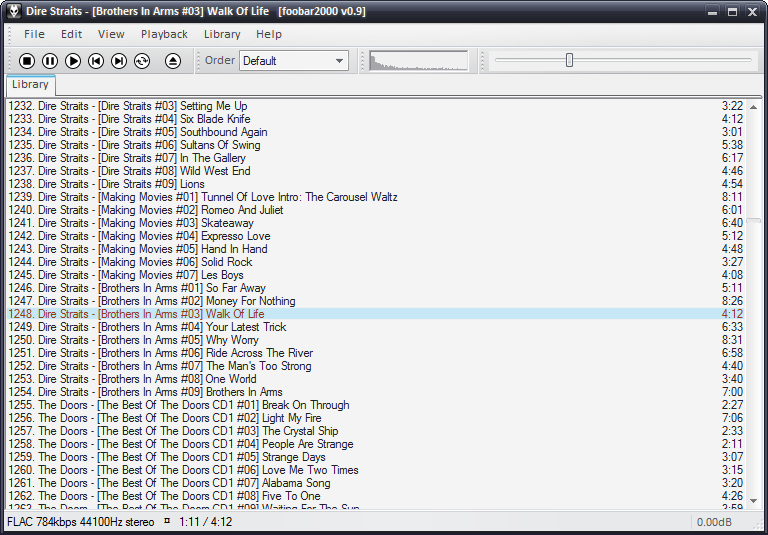Screenshot of foobar2000 v0.9 using the default user interface
Developer(s) Peter Pawlowski
Release information
Initial release {{{released}}}
Stable release TBC
Preview release {{{preview_release}}}
Compatibility
Operating system Windows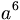$a^6$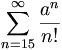$\sum_{n=15}^\infty \frac{a^n}{n!}$